### NCERT Solutions For Class 10 Math Chapter – 3 Exercise – 3.2

NCERT Solutions For Class 10 Math Chapter – 3 Linear equation in two variables Exercise – 3.2

Q1. From the pair of linear equations in the following problems, and find their solutions graphically.

• 10 students of Class 10 took part in a Mathematics quiz. If the number of girls is 4 more than the number of boys, find the number of boys and girls who took part in the quiz.

Solution:-

Let the number of boys = x

No of girls = y

Given that total number of student is 10 so that

X + y = 10

Subtract y both side we get

X = 10 – y

Putting

y = 0 , 5 , 10 we get

X = 10 – 0

X = 10 – 5 = 5

X = 10 – 10 = 0

 x 10 5 y 0 5

Given that if the number of girls is 4 more than the number of boys

So that

Y = x + 4

Putting x = -4 , 0 , 4 and we get

Y = -4 + 4 = 0

Y = 0 + 4  = 4

Y= 4 + 4 = 8

 x -4 0 4 y 0 4 8

Graphical representation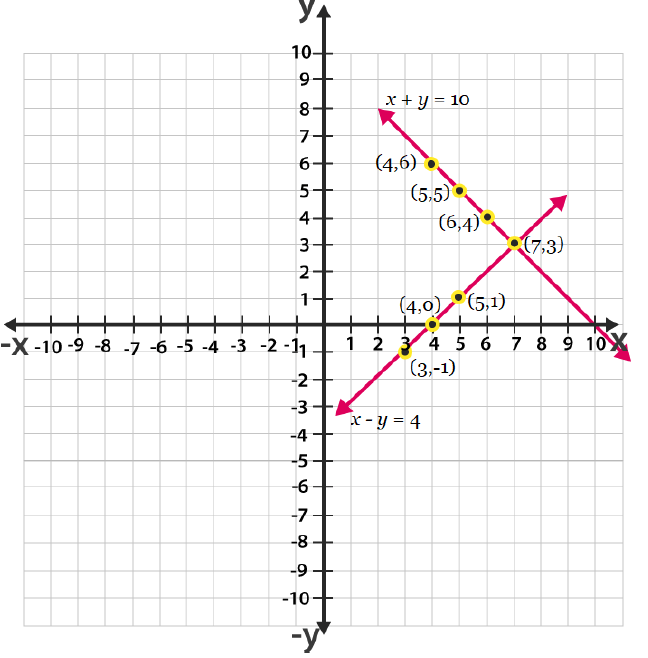Therefore , number of boys = 3 and number of girls = 7.

• 5 pencils and 7 pens together cost Rs 50 whereas 7 pencils and 5 pens together cost  Rs 46 . Find the cost of one  pencil and that of one pen.

Solution:-

5x + 7y = 50

5x = 50 – 7y

X = 10 – 7y/5

Putting value of y = 5 , 10 and 15 we get

X = 10 — 7 x 5/5 = 10 – 7 = 3

X = 10 – 7 x 10/5 = 10 – 14 = -4

X = 10 – 7 x 15/5 = 10 – 21 = 11

 x 3 -4 -11 y 5 10 15

7x + 5y = 46

5y = 46 – 7x

Y= 9.2 – 1.4x

Putting x = 0.2 and 4 we get

Y = 9.2 – 1.4 x 0 = 9.2

Y = 9.2 – 1.4 x 2 = 6.4

Y = 9.2 – 1.4 (4) = 3.6

 x 0 2 4 y 9.2 6.4 3.6

Graphical representation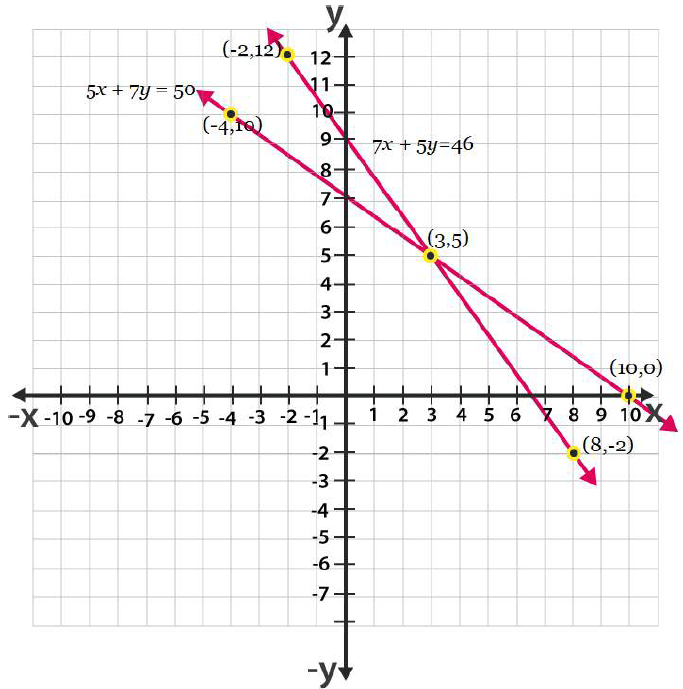Therefore , cost of one pencil = Rs 3 and cost of cost of one pen = Rs 5.

Q2. On comparing the ratios a1/a2 , b1/b2 and c1/c2 find out whether the lines representing the following pairs of linear equations intersect at a point, are parallel or  coincident.

Solution:-

• 5x – 4y + 8 = 0

7x + 6y – 9 = 0

Therefore , both are intersecting lines at one point.

• 9x + 3y + 12 = 0

18x + 6y + 24 = 0

Therefore , both lines are coincident.

• 6x – 3y + 10 = 0

2x – y + 9 = 0

Therefore , both lines are parallel.

Q3. On comparing the ratios a1/a2 , b1/  and c1/c2 find out whether the following pair of linear equations are consistent , or inconsistent.

• 3x + 2y = 5 ; 2x – 3y = 7
• 2x – 3y = 8 ; 4x – 6y = 9
• 3/2x + 5/3y = 7 ; 9x –  10y = 14
• 5x – 3y = 11 ;  -10x + 6y = -22
• 4/3x + 2y = 8 ; 2x + 3y = 12

Solution:-

• Hence pair of linear equation is consistent.
• Hence the pair of linear equation is inconsistent.
• Hence the pair of linear equation is consistent.
• Hence the pair of linear equation is consistent
• Hence the pair of linear equation is consistent.

Q4.  Which  of the following pairs of linear equation are consistent/inconsistent ? If consistent, obtain  the solution graphically;

• X + y = 5 , 2x + 2y = 10
• X – y = 8 , 3x – 3y = 16
• 2x + y – 6 = 0 , 4x – 2y – 4 = 0
• 2x – 2y – 2 = 0, 4x – 4y – 5 = 0

Solution:-

• X + y = 5 ; 2x + 2y = 10

Hence the pair of linear equation is consistent.

X + y = 5

X = 5 – y

 x 4 3 2 y 1 2 3

And , 2x + 2y = 10

X = 10 – 2y/2

 x 4 3 2 y 1 2 3

Graphical representation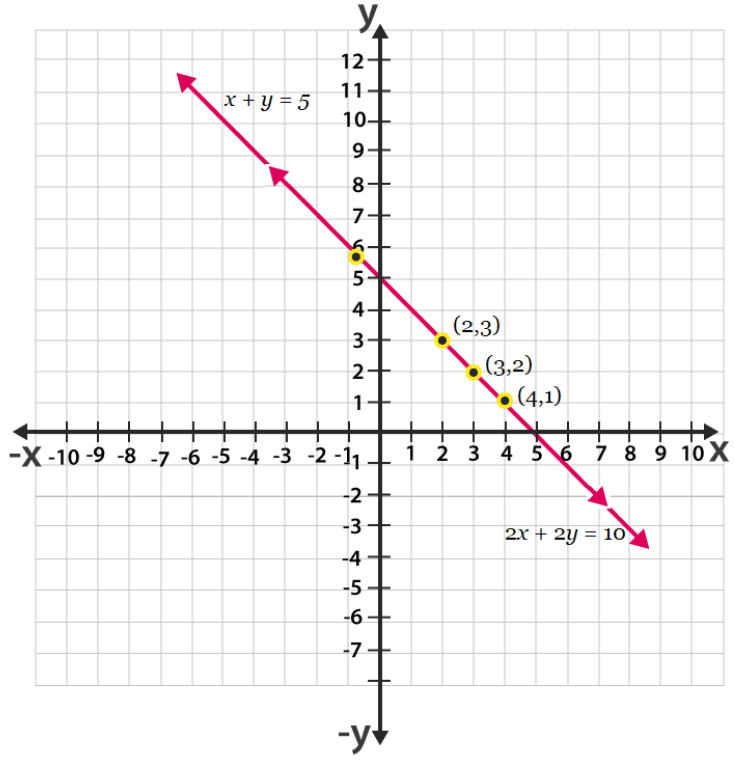From the figure it can be observed that these lines are overlapping each other. Therefore , infinite solutions are possible for the given pair of equations.

• X – y = 8 , 3x – 3y = 16

Hence the pair of linear equation is inconsistent.

• 2x + y – 6 = 0  , 4x – 2y – 4 = 0

Hence the pair of linear equation is consistent.

2x + y – 6 = 0

Y = 6 – 2x

 x 0 1 2 y 6 4 2

And , 4x – 2y – 4 = 0

Y = 4x – 4/2

 x 1 2 3 y 0 2 4

Graphical representation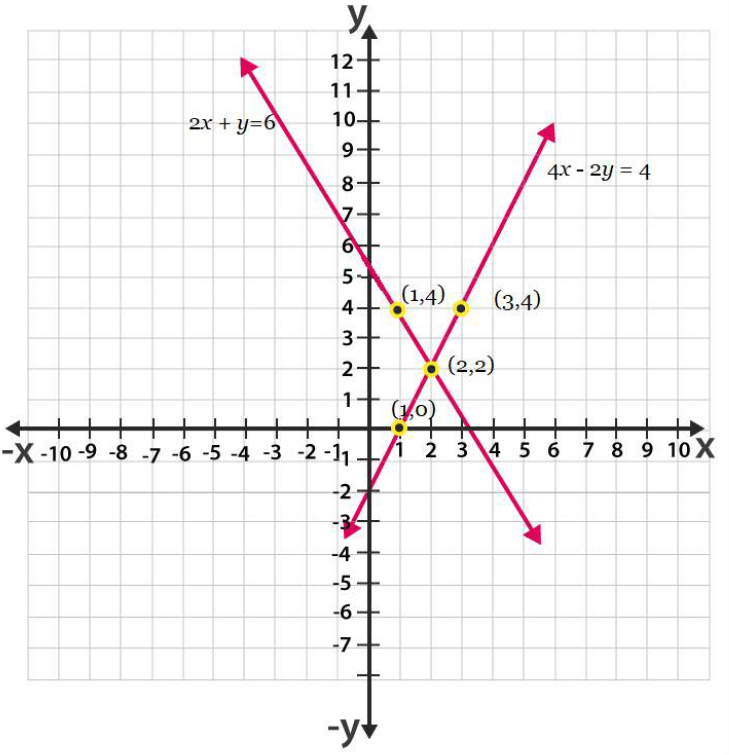From the figure , it can be observed that these lines are intersecting each other at the only one point (2,2) which is the solution for the given pair of equations.

• 2x – 2y – 2 = 0 ,  4x – 4y – 5 = 0

Hence the pair of linear equation is inconsistent

Q5. Half the perimeter of a rectangular garden , whose length is 4 m more than its width, is 36 m. Find the dimensions of the garden.

Solution:-

Y – x = 4 (i)

Y + x = 36 (ii)

Y – x = 4

Y = x + 4

 x 0 8 12 y 4 12 16

Y + x = 36

 x 0 36 16 y 36 0 20

Graphical representation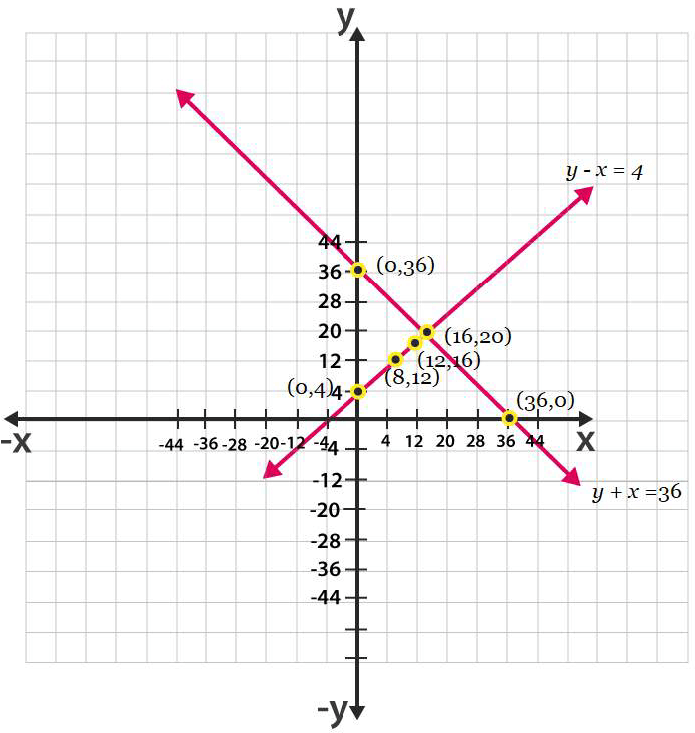From the figure , it can be observed that these lines are intersecting each other at only point (16,20) Therefore , the length and width of the given garden is 20 m and 16  m respectively.

Q6. Given the linear equation 2x + 3y – 8 = 0 write another linear equations in two variables such that the geometrical representation of the pair so formed is:

• Intersecting lines
• Parallel lines
• Coincident lines

Solution:-

• Intersecting lines:

For this conditions

A1/a2 ≠ b1/b2

• Parallel lines

For this conditions

A1/a2 = b1/b2 ≠ c1 / c2

• Coincident lines

For coincident lines

A1/a2 = b1/b2 = c1/c2

Q7. Draw the graph of the equations x – y + 1 = 0 and 3x + 2y – 12 = 0 . Determine the coordinates of the vertices of the triangle formed by these lines and the x – axis , and shade the triangular region.

Solution:-

X – y + 1 = 0

X = y – 1

 x 0 1 2 y 1 2 3

3x + 2y – 12 = 0

X = 12 – 2y/3

 x 4 2 0 y 0 3 6

Graphical  representation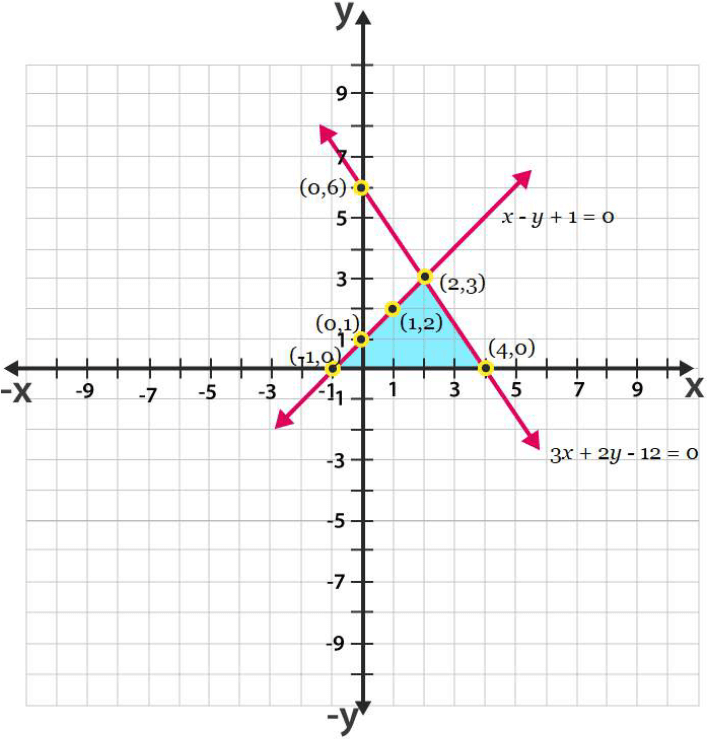From the figure it can be observed that these lines are intersecting each other at point (2,3) and x – axis at (1,0) and (4,0) Therefore , the vertices of the triangle are (2,3) (-1,0) and (4,0).

NCERT Solutions For Class 10 Math Chapter – 3 Linear equation in two variables Exercise – 3.2   Q1. From the…